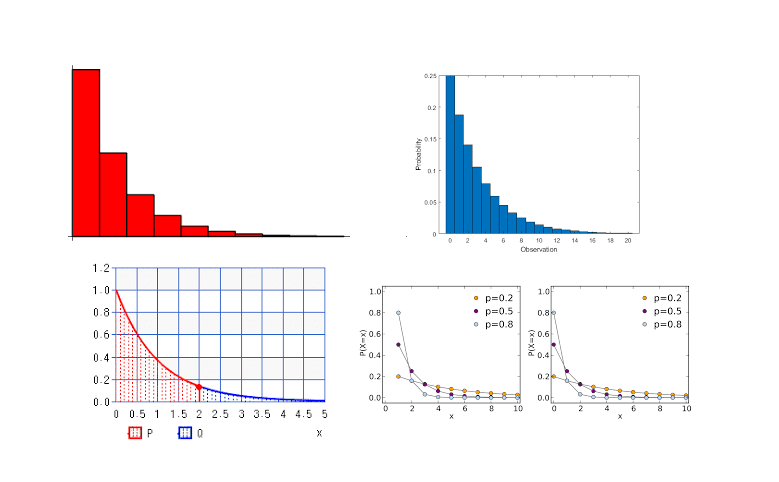Get inspired by the success stories of our students in IIT JAM MS, ISI  MStat, CMI MSc Data Science.  Learn More

# Testing of Hypothesis | ISI MStat 2016 PSB Problem 9This is a problem from the ISI MStat Entrance Examination, 2016 involving the basic idea of Type 1 error of Testing of Hypothesis but focussing on the fundamental relationship of Exponential Distribution and the Geometric Distribution.

## The Problem:

Suppose $X_{1}, X_{2}, \ldots, X_{n}$ is a random sample from an exponential distribution with mean $\lambda$.

Assume that the observed data is available on $\left[X_{1}\right], \ldots,\left[X_{n}\right]$, instead of $X_{1}, \ldots, X_{n},$ where $[x]$ denotes the largest integer less than or equal to $x$.

Consider a test for $H_{0}: \lambda=1$ vs $H_{1}: \lambda>1$ which rejects $H_{0}$ when $\sum_{i=1}^{n}\left[X_{i}\right]>c_{n} .$

Given $\alpha \in(0,1),$ obtain values of $c_{n}$ such that the size of the test converges to $\alpha$ as $n \rightarrow \infty$.

## Prerequisites:

(a) Testing of Hypothesis

(b) Type 1 Error

(c) Exponential Distribution

(d) Relationship of Exponential Distribution and Geometric Distribution

(e) Central Limit Theorem

## Solution:

• X ~ Exponential($\lambda$), then $Y = [\frac{X}{a}]$ ~ Geom($p$), where $p = 1-e^{-\lambda a} \in(0,1)$

Proof:

$Y$ is clearly discrete taking values in the set of non-negative integers, due to the flooring. Then, for any integer $n \geq 0$ we have
$\begin{array}{c} P(Y=n)=P(X \in[\text {an, } a(n+1))) \ =\int_{a n}^{a(n+1)} \lambda \mathrm{e}^{-\lambda x} d x=(1-p)^{n} p \end{array}$
where $p=1-e^{-\lambda a} \in(0,1),$ as $\lambda>0$ and $a>0$.

• $X_i$ ~ Geom($p$), then $\sum_{i = 1}^{n}$ ~ NBinom(n,p)
• $X_i$ ~ Exponential($\lambda$), then $S_n = \sum_{i=1}^{n}\left[X_{i}\right]$ ~ NBinom($(n,p)$), where $p = 1-e^{-\lambda} \in(0,1)$

#### Testing of Hypothesis

$H_{0}: \lambda=1$ vs $H_{1}: \lambda>1$

We reject $H_{0}$ when $\sum_{i=1}^{n}\left[X_{i}\right]>c_{n} .$

Here, the size of the test i.e the Type 1 error (for simple hypothesis), $\alpha_n$ = $P(S_n > c_{n} | \lambda=1)$.

We want to select $c_n$ such that $\alpha_n \to \alpha$.

$S_n$ ~ NBinom($n,p$), where $p = 1-e^{-1}$ under $H_0$.

Now, $\frac{\sqrt{n}(\frac{S_n}{n} - \frac{1}{p})}{\sqrt{\frac{1-p}{p^2}}} \rightarrow Z = N(0,1)$ by Central Limit Theorem.

Observe that thus, $\alpha_n = P(S_n > c_{n} | \lambda=1) \rightarrow P(Z > \frac{\sqrt{n}(\frac{c_n}{n} - \frac{1}{p})}{\sqrt{\frac{1-p}{p^2}}}) = \alpha$.

Thus, $\frac{\sqrt{n}(\frac{c_n}{n} - \frac{1}{p})}{\sqrt{\frac{1-p}{p^2}}} = Z_{\alpha}$.

We can solve this to find $c_n$, where $p = 1-e^{-1}$

## Food for Thought

If X ~ Exponential($\lambda$), then what is the distribution of {X} [ The fractional part of X]. This question is crucial is getting back Exponential Distrbution from Geometric Distribution.

Rather, the food for thought, asks you how do we get Exponential Distribution from Geometric Distribution.

Stay Tuned. Stay Blessed! See you in the next post.

This is a problem from the ISI MStat Entrance Examination, 2016 involving the basic idea of Type 1 error of Testing of Hypothesis but focussing on the fundamental relationship of Exponential Distribution and the Geometric Distribution.

## The Problem:

Suppose $X_{1}, X_{2}, \ldots, X_{n}$ is a random sample from an exponential distribution with mean $\lambda$.

Assume that the observed data is available on $\left[X_{1}\right], \ldots,\left[X_{n}\right]$, instead of $X_{1}, \ldots, X_{n},$ where $[x]$ denotes the largest integer less than or equal to $x$.

Consider a test for $H_{0}: \lambda=1$ vs $H_{1}: \lambda>1$ which rejects $H_{0}$ when $\sum_{i=1}^{n}\left[X_{i}\right]>c_{n} .$

Given $\alpha \in(0,1),$ obtain values of $c_{n}$ such that the size of the test converges to $\alpha$ as $n \rightarrow \infty$.

## Prerequisites:

(a) Testing of Hypothesis

(b) Type 1 Error

(c) Exponential Distribution

(d) Relationship of Exponential Distribution and Geometric Distribution

(e) Central Limit Theorem

## Solution:

• X ~ Exponential($\lambda$), then $Y = [\frac{X}{a}]$ ~ Geom($p$), where $p = 1-e^{-\lambda a} \in(0,1)$

Proof:

$Y$ is clearly discrete taking values in the set of non-negative integers, due to the flooring. Then, for any integer $n \geq 0$ we have
$\begin{array}{c} P(Y=n)=P(X \in[\text {an, } a(n+1))) \ =\int_{a n}^{a(n+1)} \lambda \mathrm{e}^{-\lambda x} d x=(1-p)^{n} p \end{array}$
where $p=1-e^{-\lambda a} \in(0,1),$ as $\lambda>0$ and $a>0$.

• $X_i$ ~ Geom($p$), then $\sum_{i = 1}^{n}$ ~ NBinom(n,p)
• $X_i$ ~ Exponential($\lambda$), then $S_n = \sum_{i=1}^{n}\left[X_{i}\right]$ ~ NBinom($(n,p)$), where $p = 1-e^{-\lambda} \in(0,1)$

#### Testing of Hypothesis

$H_{0}: \lambda=1$ vs $H_{1}: \lambda>1$

We reject $H_{0}$ when $\sum_{i=1}^{n}\left[X_{i}\right]>c_{n} .$

Here, the size of the test i.e the Type 1 error (for simple hypothesis), $\alpha_n$ = $P(S_n > c_{n} | \lambda=1)$.

We want to select $c_n$ such that $\alpha_n \to \alpha$.

$S_n$ ~ NBinom($n,p$), where $p = 1-e^{-1}$ under $H_0$.

Now, $\frac{\sqrt{n}(\frac{S_n}{n} - \frac{1}{p})}{\sqrt{\frac{1-p}{p^2}}} \rightarrow Z = N(0,1)$ by Central Limit Theorem.

Observe that thus, $\alpha_n = P(S_n > c_{n} | \lambda=1) \rightarrow P(Z > \frac{\sqrt{n}(\frac{c_n}{n} - \frac{1}{p})}{\sqrt{\frac{1-p}{p^2}}}) = \alpha$.

Thus, $\frac{\sqrt{n}(\frac{c_n}{n} - \frac{1}{p})}{\sqrt{\frac{1-p}{p^2}}} = Z_{\alpha}$.

We can solve this to find $c_n$, where $p = 1-e^{-1}$

## Food for Thought

If X ~ Exponential($\lambda$), then what is the distribution of {X} [ The fractional part of X]. This question is crucial is getting back Exponential Distrbution from Geometric Distribution.

Rather, the food for thought, asks you how do we get Exponential Distribution from Geometric Distribution.

Stay Tuned. Stay Blessed! See you in the next post.

This site uses Akismet to reduce spam. Learn how your comment data is processed.

### Knowledge Partner